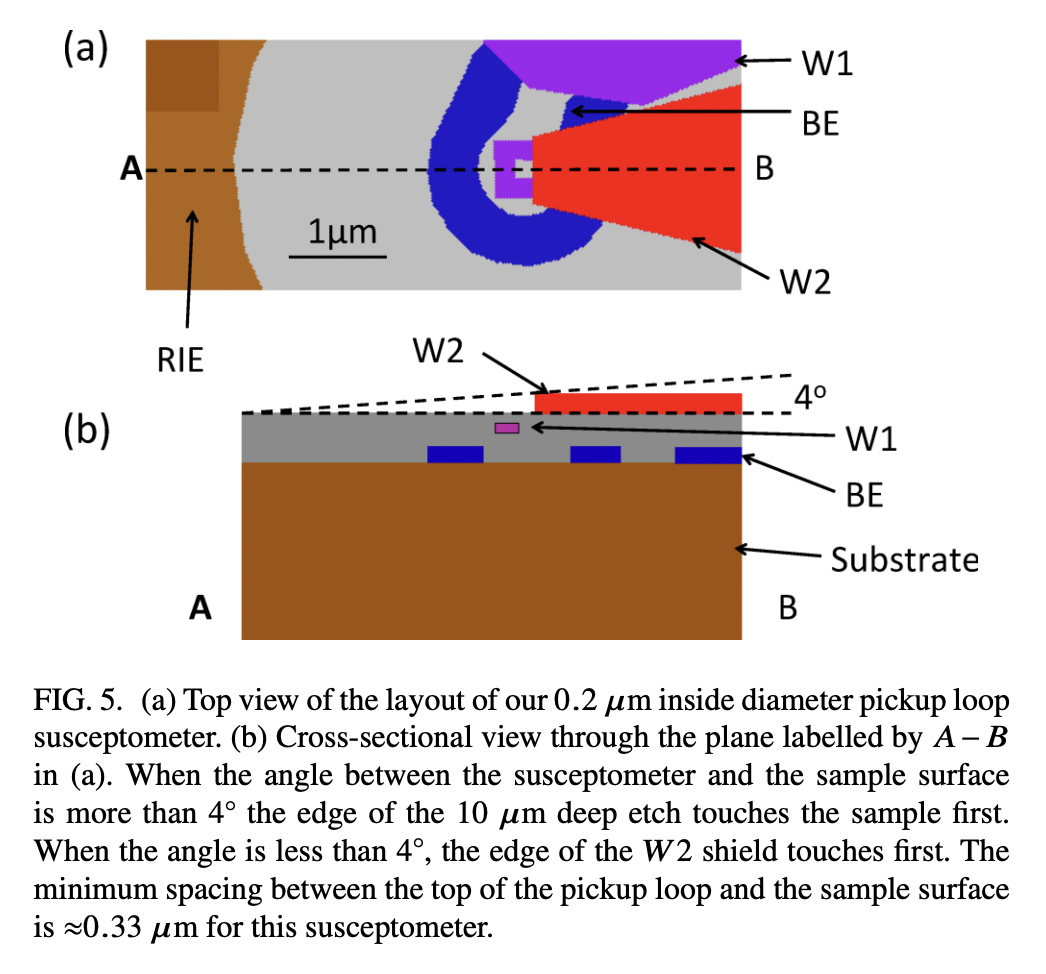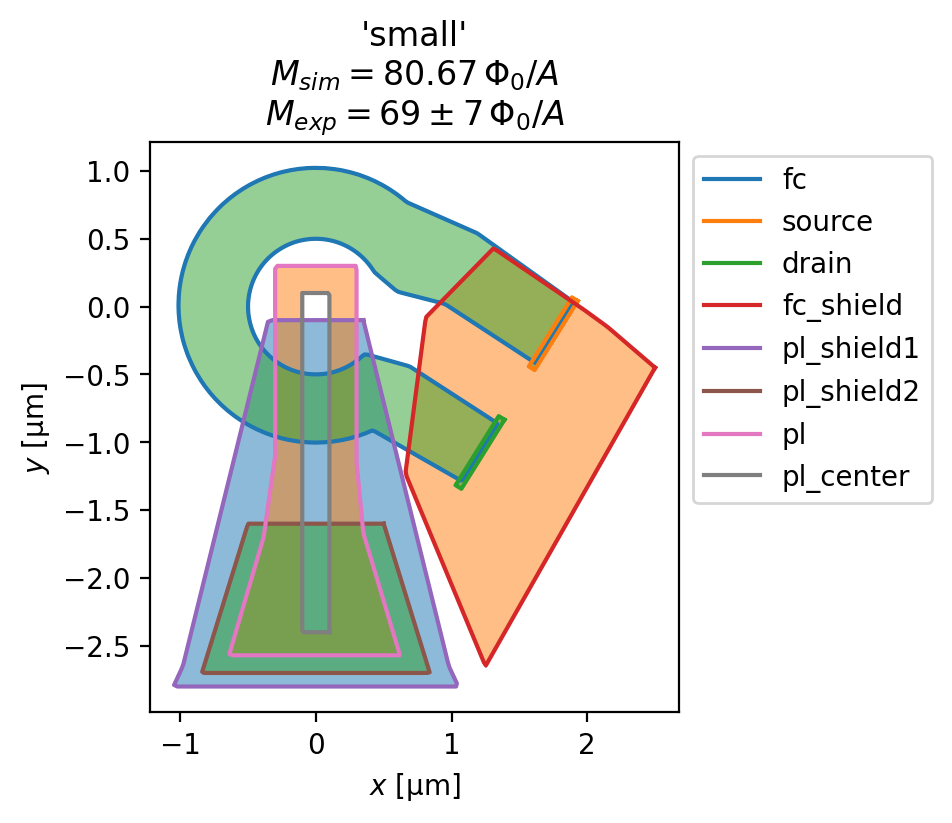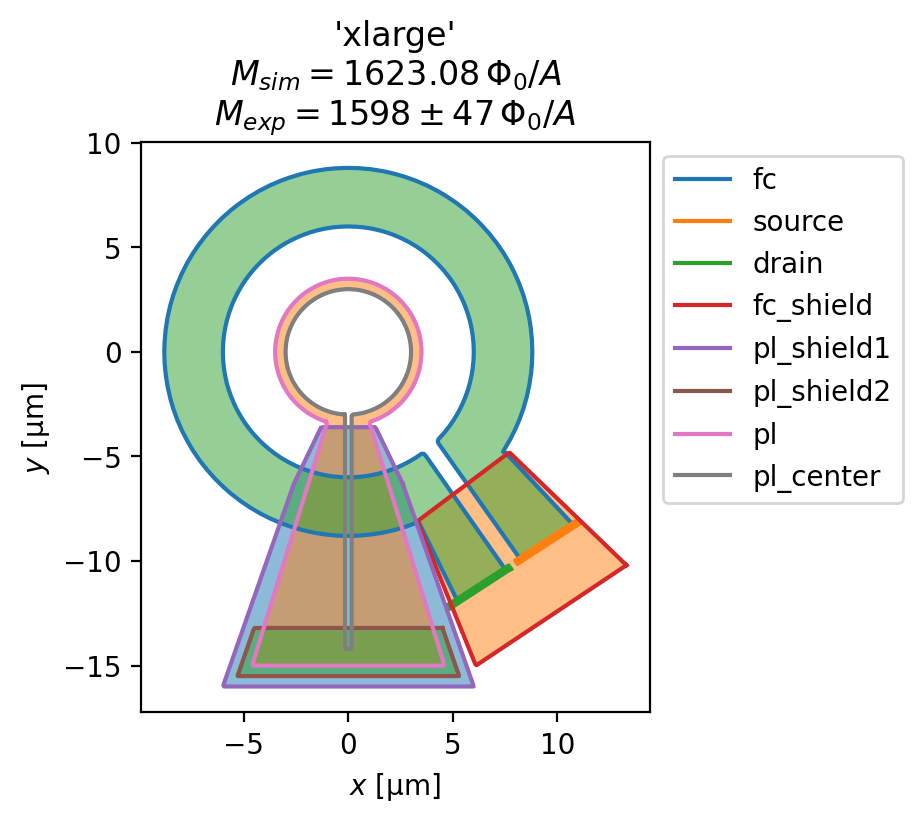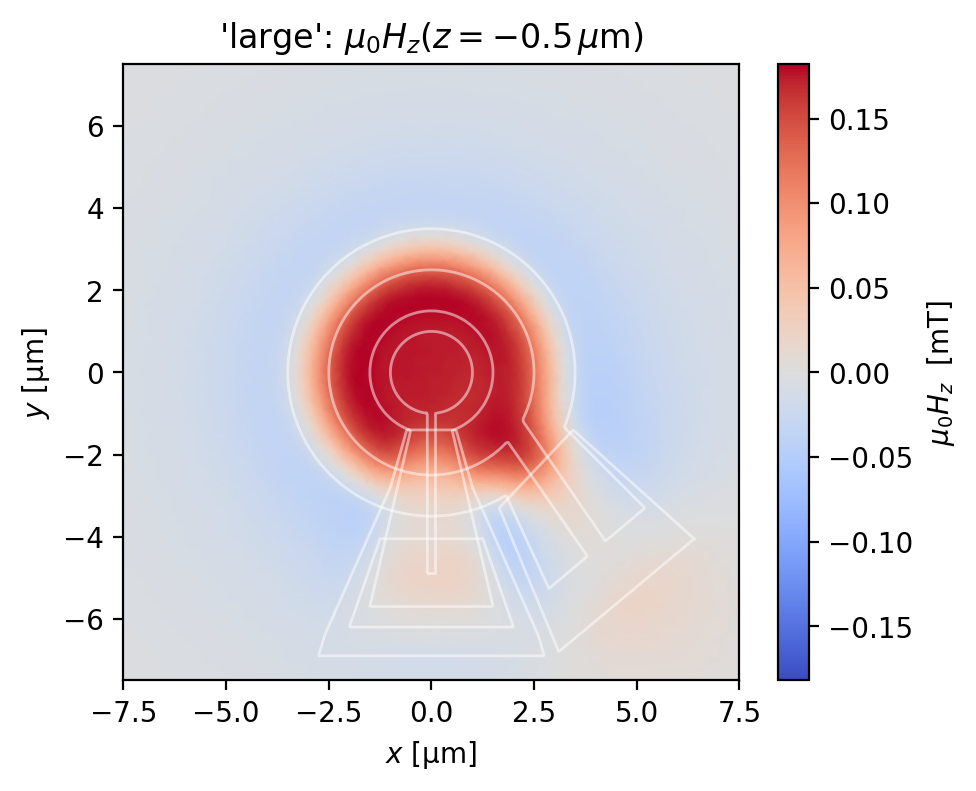# Scanning SQUID microscopy

One of the original motivations for SuperScreen was to model scanning superconducting quantum interference device (SQUID) magnetometers/susceptometers used in scanning SQUID microscopy. In this notebook we demonstrate how SuperScreen can be used to calculate the mutual inductance between the field coil and pickup loop in state-of-the-art scanning SQUID susceptometers (Rev. Sci. Instrum. 87, 093702 (2016), arXiv:1605.09483).

The layouts for four designs of scannning SQUID susceptometer are shown below (taken from Figure 4 of Kirtley, et al., Scanning SQUID susceptometers with sub-micron spatial resolution, Rev. Sci. Instrum. 87, 093702 (2016)).There are three relevant superconducting wiring layers, labeled “BE” (blue), “W1” (purple), and “W2” (red). The pickup loop, the flux-sennsing loop that is part of the SQUID circuit, sits in the purple “W1” wiring layer. The pickup loop is partially covered by a superconducting shield in the red “W2” wiring layer, which sits between the pickup loop and the sample being measured. A single-turn field coil sitting in the blue “BE” wiring layer can be used to locally apply a magnetic field to the sample. The layer structure of the SQUID susceptometers is shown below (taken from Figure 5 of Kirtley, et al., Scanning SQUID susceptometers with sub-micron spatial resolution, Rev. Sci. Instrum. 87, 093702 (2016)).The pickup loop and field coil can be used to perform a mutual inductance AC susceptibility measurement in a reflection geometry. The presence of a paramagnetic or diamagnetic sample near the pickup loop and field coil modifies the mutual inductance between the two loops, and the strength of the modification is a measure of the magnetic susceptibility of the sample. Quantitative modeling of the magnetic response of such multi-layer superconducting circuits is important both for interpreting measurements and for designing next-generation sensors.

The experimentally measured values of the pickup loop - field coil mutual inductance (in units of $$\Phi_0/\mathrm{A}$$, the flux induced in the pickup loop per unit current flowing in the field coil) are shown below. The value are taken from Table 1 of Rev. Sci. Instrum. 87, 093702 (2016). As shown below, we find excellent agreement between SuperScreen models derived from the as-designed SQUID layouts and the experimentally measured mutual inductance.

:

exp_mutuals = {
"small": (69, 7),  # panel (a) in the first figure
"medium": (166, 4),  # panel (b)
"large": (594, 24),  # panel (c)
"xlarge": (1598, 47),  # panel (d)
}

:

# Automatically install superscreen from GitHub only if running in Google Colab
%pip install --quiet git+https://github.com/loganbvh/superscreen.git

:

%config InlineBackend.figure_formats = {"retina", "png"}
%matplotlib inline

import os
import sys

import matplotlib.pyplot as plt

plt.rcParams["figure.figsize"] = (5, 4)
plt.rcParams["font.size"] = 10

import superscreen as sc
from superscreen.geometry import box

sys.path.insert(0, "..")
import squids

:

sc.version_table()

:

SoftwareVersion
SuperScreen0.10.5
Numpy1.26.1
Numba0.58.1
SciPy1.11.3
matplotlib3.8.1
IPython8.17.2
Python3.9.18 (main, Oct 18 2023, 22:10:26) [GCC 9.3.0]
OSposix [linux]
Number of CPUsPhysical: 1, Logical: 2
BLAS InfoGeneric
Fri Nov 03 18:09:57 2023 UTC

## Define and solve the models

:

squid_funcs = {
"small": squids.ibm.small.make_squid,
"medium": squids.ibm.medium.make_squid,
"large": squids.ibm.large.make_squid,
"xlarge": squids.ibm.xlarge.make_squid,
}
mesh_kwargs = {
"small": dict(max_edge_length=0.1, smooth=100),
"medium": dict(max_edge_length=0.1, smooth=100),
"large": dict(max_edge_length=0.15, smooth=100),
"xlarge": dict(max_edge_length=0.4, smooth=100),
}


Here, we simulate the reponse of the four SQUID susceptometers to a fixed current of 1 mA flowing counterclockwise in the field coil.

:

solutions = {}
I_fc = "1 mA"
for name, make_squid in squid_funcs.items():
squid = make_squid()
squid.make_mesh(**mesh_kwargs[name])
solutions[name] = sc.solve(
squid,
terminal_currents={"fc": {"source": I_fc, "drain": f"-{I_fc}"}},
iterations=5,
)[-1]

Solver iterations: 100%|██████████| 5/5 [00:07<00:00,  1.47s/it]
Solver iterations: 100%|██████████| 5/5 [00:07<00:00,  1.56s/it]
Solver iterations: 100%|██████████| 5/5 [00:27<00:00,  5.58s/it]
Solver iterations: 100%|██████████| 5/5 [00:22<00:00,  4.42s/it]


## Evaluate the pickup loop - field coil mutual inductance

:

for name, solution in solutions.items():
squid = solution.device
fig, ax = squid.draw()
_ = squid.plot_polygons(ax=ax, legend=True)

fluxoid = sum(solution.hole_fluxoid("pl_center"))
mutual_inductance = (fluxoid / sc.ureg(I_fc)).to("Phi_0 / A").magnitude
mean, rng = exp_mutuals[name]
title = [
f"{name!r}",
rf"$M_{{sim}}={{{mutual_inductance:.2f}}}\,\Phi_0/A$",
rf"$M_{{exp}}={{{mean}}}\pm{{{rng}}}\,\Phi_0/A$"
]
ax.set_title("\n".join(title))## Evaluate the magnetic field generated by the susceptometer

Below, we plot the $$z$$-component of the magnetic field generated by the SQUID susceptometer field coil, evaluated at a plane 0.5 $$\mu$$m from the top wiring layer of the susceptometer.

:

eval_regions = {
# name: (width, height, z-position)
"small": (5, 5, -0.5),
"medium": (7.5, 7.5, -0.5),
"large": (15, 15, -0.5),
"xlarge": (25, 25, -0.5),
}
for name, solution in solutions.items():
width, height, z = eval_regions[name]
eval_mesh = sc.Polygon(points=box(width, height, points=201)).make_mesh(min_points=4000)
fig, ax = solution.plot_field_at_positions(
eval_mesh,
zs=z,
symmetric_color_scale=True,
cmap="coolwarm",
)
squid = solution.device
for polygon in squid.get_polygons(include_terminals=False):
polygon.plot(ax=ax, color="w", lw=1, alpha=0.5)
ax.set_title(rf"{name!r}: $\mu_0H_z(z={{{z}}}\,\mu\mathrm{{m}})$")[ ]: